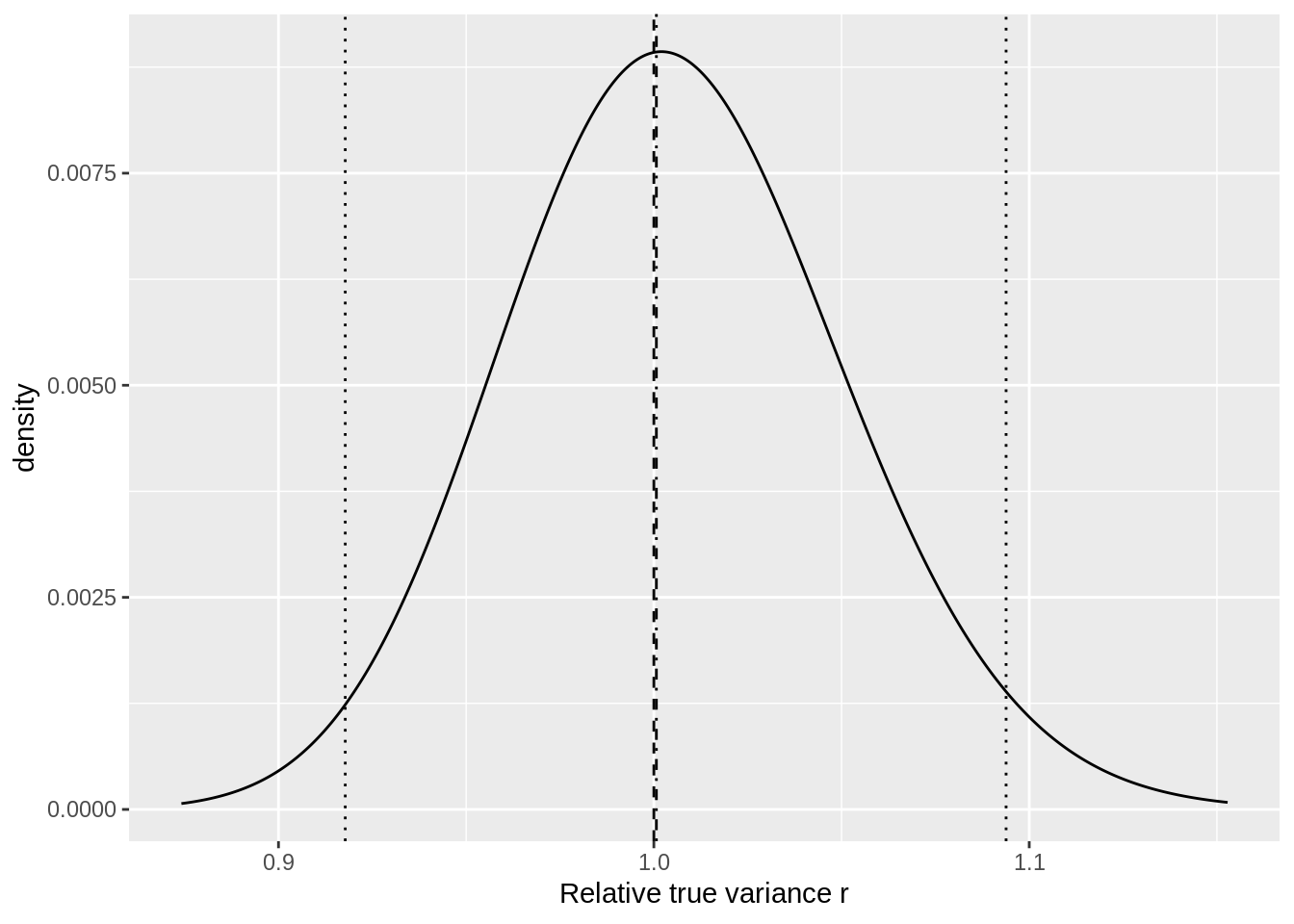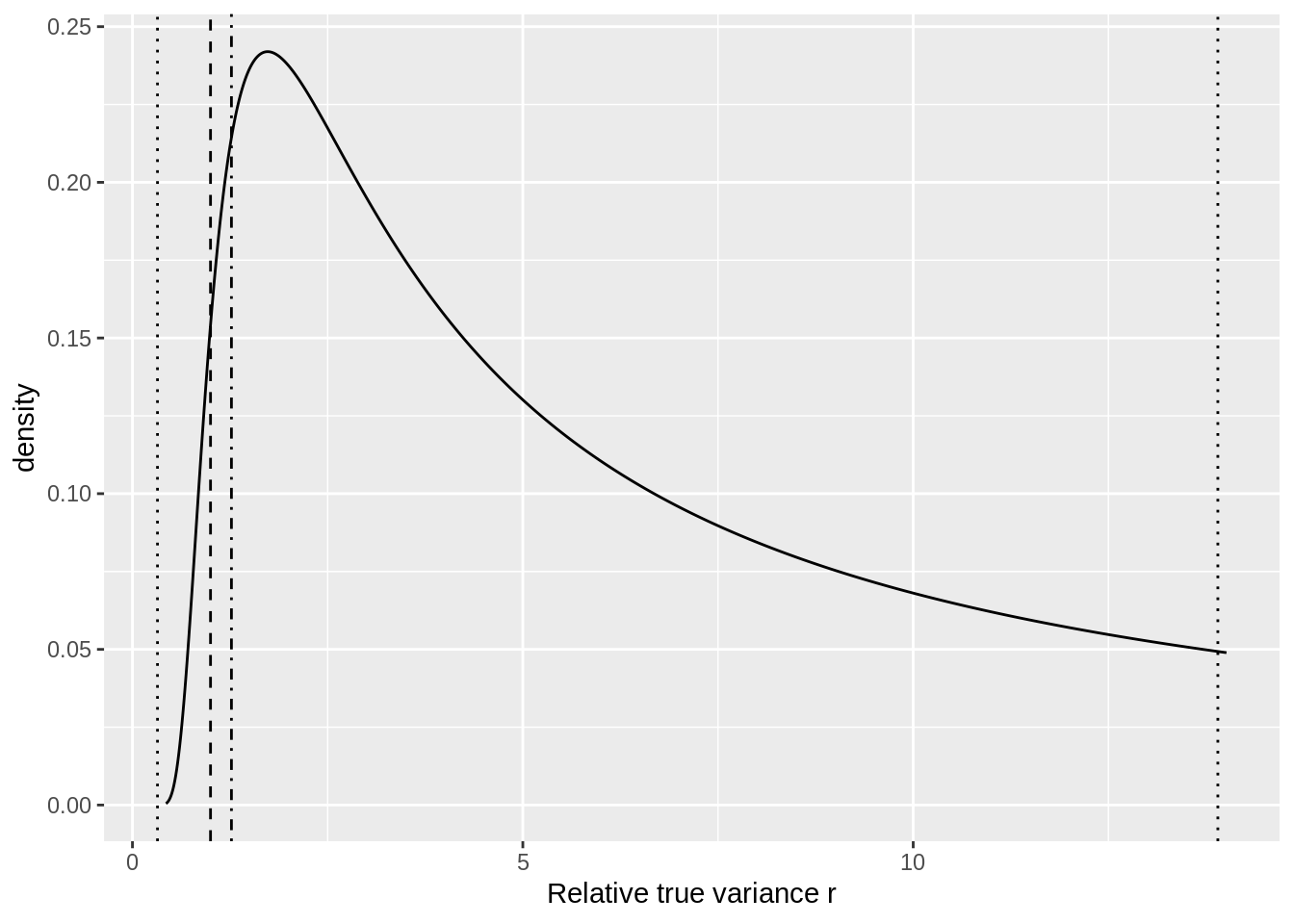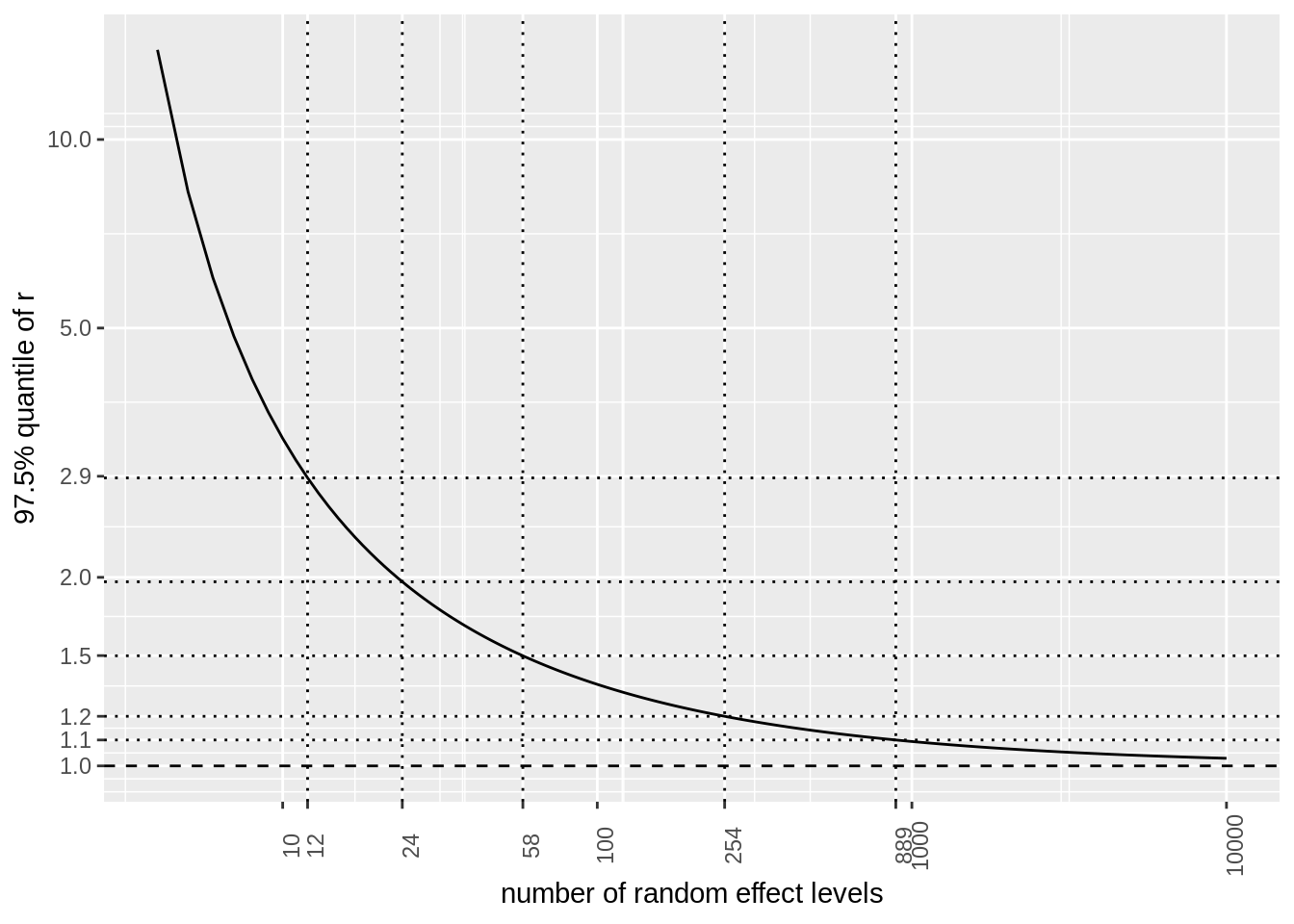# Required number of levels for a random effects

When we analyse a mixed models, the question often arises whether a covariate should be used as a random effect or as a fixed effects. Let’s assume a simple design. Two types of fertilizer are tested on a number of fields ($n_s$). Each field is split in two and the fertilizers are assigned at random to these halves while making sure that each field has both treatments. From a conceptual point of view, we are only interested in the effect of the fertilizers. However, we need to take the potential effect of the field into account. Hence we use fertiliser as a fixed effect and field as a random effect.

Table 1: Example design
field Part 1 Part2
1 A B
2 A B
3 B A
4 B A
5 A B
6 B A
7 A B
8 A B
9 B A
10 A B

While this makes conceptually sense, we might run into computational problems. Instead of estimating each individual field effect, we want to estimate the variability due to field effect. This is the variance of the random effect, rather than the estimates for the individual random effect levels. But how precise is the estimate of this random effect variance? The sample variance $s^2$ follows a scaled $\chi^2$ distribution.

$(n_s - 1)\frac{s^2}{\sigma^2} \sim \chi^2_{n_s-1}$

This equation makes it straightforward to calculate the distribution of the $r = \sigma^2/s^2$ ratio for a given $n_s$. We can rewrite this as $\sigma^2=rs^2$ or the true variance is a multiple $r$ of the estimated variance $s^2$. The distribution of $r$ is derived below.

$(n_s - 1)\frac{s^2}{\sigma^2} \sim \chi^2_{n_s-1}$

$\frac{s^2}{\sigma^2} \sim \frac{\chi^2_{n_s-1}}{(n_s - 1)}$

$r = \frac{\sigma^2}{s^2} \sim \frac{(n_s - 1)}{\chi^2_{n_s-1}}$

Even when with a large number of levels ($n_s = 1000$), there still is a reasonable amount of uncertainty around $\sigma^2$ since the 95% interval of $r$ ranges from 0.918 to 1.094. The full distribution for $r$ when $n_s = 1000$ is shown in the figure below.Figure 1: Distribution of the relative true variance ($\frac{\sigma^2}{s^2}$) when $n_s = 1000$. Dashed line: $\frac{\sigma^2}{s^2} = 1$ Dotted lines: 2.5% and 97.5% quantiles of $\frac{\sigma^2}{s^2}$. Dash-dotted line: median of $\frac{\sigma^2}{s^2}$.

A thousand random effect levels is not always feasible. So what will happen if we use a more realistic number of random effect levels, e.g. $n_s = 20$. The distribution of $r$ will be wider as $n_s$ decreases. At $n_s = 20$, the 95% interval of $r$ will range from 0.578 to 2.133.Figure 2: Distribution of the relative true variance ($\frac{\sigma^2}{s^2}$) when $n_s = 20$. Dashed line: $\frac{\sigma^2}{s^2} = 1$ Dotted lines: 2.5% and 97.5% quantiles of $\frac{\sigma^2}{s^2}$. Dash-dotted line: median of $\frac{\sigma^2}{s^2}$.

How low can we go? The figure below depicts the density of $r$ when $n_s = 4$. The 95% interval of $r$ ranges from 0.321 to 13.902, so we can hardly do any inference on the estimated variance.Figure 3: Distribution of the relative true variance ($\frac{\sigma^2}{s^2}$) when $n_s = 4$. Dashed line: $\frac{\sigma^2}{s^2} = 1$ Dotted lines: 2.5% and 97.5% quantiles of $\frac{\sigma^2}{s^2}$. Dash-dotted line: median of $\frac{\sigma^2}{s^2}$.

## Recommendations

We see that the number of random effect levels has a strong impact on the uncertainty of the estimate variance. The figure below displays the 97.5% quantile of $r$ for a large range of $n_s$ values. Since the right tail of $r$ is heavier than the left tail, it is a good idea to focus on the 97.5% quantile. Based on this figure we can give the following recommendations:

• get $n_s > 1000$ levels when an accurate estimate of the random effect variance is crucial. E.g. when a single number will be use for power calculations.
• get $n_s > 100$ levels when a reasonable estimate of the random effect variance is sufficient. E.g. power calculations with sensitivity analysis of the random effect variance.
• get $n_s > 20$ levels for an experimental study
• in case $10 < n_s < 20$ you should validate the model very cautious before using the output
• in case $n_s < 10$ it is safer to use the variable as a fixed effect.